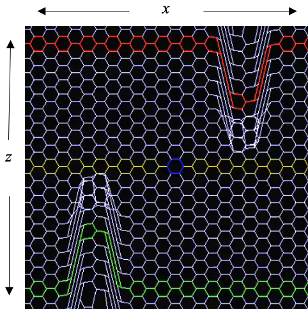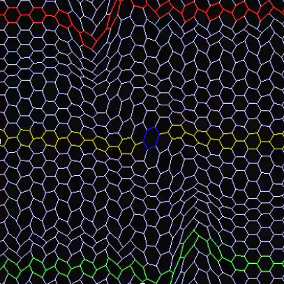## Gravitational Wave Generator Background Theory

Foamy Ether Theory describes electromagnetic waves as distortions in the foam that are transverse to the direction of propagation. When two electromagnetic waves, traveling in opposite directions meet, a compression of foamy ether (space) takes place between the waves. Screen captures of a simulation (using ThreeDimSim) of this compression is illustrated in Figure 1.

Figure1a shows the initial wave where the red wave on top is traveling from right to left and the green wave on the bottom is traveling from left to right. When the two waves are aligned, as shown in Figure 1b, a compression of foamy ether takes place momentarily (see the blue cell in the center of Figure1b). As the waves leave each other (Figure 1c), a momentary stretching of the foam occurs. This compressing and stretching is equivalent to a gravitational wave which will propagate into the surrounding space.

Figure 2 shows the full simulation.Figure 1aFigure 1bFigure 1cFigure 2

Figure 3 below is a plot of the distortions in the foamy ether that take place as the EM waves (from Figure 1) pass by each other. The green and red lines show a maximum distortion of +40 and -40 units respectively in the z direction. The blue line plots the changes in height (z direction) of the blue cell in the middle as it goes through the compression and stretching. It compresses by a maximum of 12 units at time 19 and then stretches by 7 units at time 24.Figure 3

A full description of the invention of a Gravitational Wave Generator can be found on my ResearchGate page:

https://www.researchgate.net/publication/351884132_Design_for_a_Gravitational_Wave_Generator